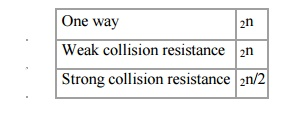Home | | Cryptography and Network Security | Security of Hash Functions and Macs

# Security of Hash Functions and Macs

Just as with symmetric and public-key encryption, we can group attacks on hash functions and MACs into two categories: brute-force attacks and cryptanalysis.

Security of Hash Functions and Macs

Just as with symmetric and public-key encryption, we can group attacks on hash functions and MACs into two categories: brute-force attacks and cryptanalysis.

Brute-Force Attacks

The nature of brute-force attacks differs somewhat for hash functions and MACs.

Hash Functions

The strength of a hash function against brute-force attacks depends solely on the length of the hash code produced by the algorithm. Recall from our discussion of hash functions that there are three desirable properties:

·        One-way: For any given code h, it is computationally infeasible to find x such that H(x) = h.

·        Weak collision resistance: For any given block x, it is computationally infeasible to find y x with H(y) = H(x).

·        Strong collision resistance: It is computationally infeasible to find any pair (x, y) such that H(x) = H(y).

·        For a hash code of length n, the level of effort required, as we have seen is proportional to the following:Message Authentication Codes

A brute-force attack on a MAC is a more difficult undertaking because it requires known message-MAC pairs.. To attack a hash code, we can proceed in the following way. Given a fixed message x with n-bit hash code h = H(x), a brute-force method of finding a collision is to pick a random bit string y and check if H(y) = H(x). The attacker can do this repeatedly off line. To proceed, we need to state the desired security property of a MAC algorithm, which can be expressed as follows:

Computation resistance: Given one or more text-MAC pairs (xi, CK[xi]), it is computationally infeasible to compute any text-MAC pair (x, CK( x)) for any new input x ≠xi.

In other words, the attacker would like to come up with the valid MAC code for a given message x. There are two lines of attack possible: Attack the key space and attack the MAC value. We examine each of these in turn.

To summarize, the level of effort for brute-force attack on a MAC algorithm can be expressed as min(2k, 2n). The assessment of strength is similar to that for symmetric encryption algorithms. It would appear reasonable to require that the key length and MAC length satisfy a relationship such as min(k, n) ≥N, where N is perhaps in the range of 128 bits.

Cryptanalysis

As with encryption algorithms, cryptanalytic attacks on hash functions and MAC algorithms seek to exploit some property of the algorithm to perform some attack other than an exhaustive search.

Study Material, Lecturing Notes, Assignment, Reference, Wiki description explanation, brief detail
Cryptography and Network Security : Security of Hash Functions and Macs |